# Lowest Common Ancestor

Update 14-Jun-2007 12:33:13 CEST

## Fast Intel Implementation

What is Lowest Common Ancestor? I believe one image is worth more than thousand words.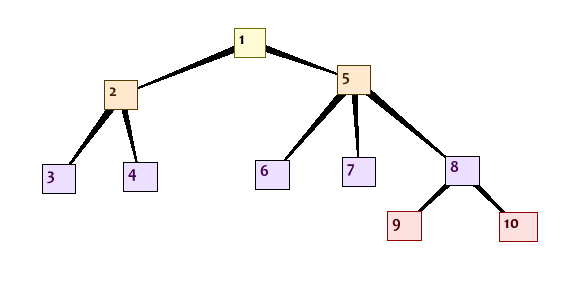LCA of node 9 and 10 is 8.
LCA of node 9 and 7 is 5.
LCA of node 8 and 4 is 1.
Now let's formalize that.
Definition - Ancestor
In a rooted tree node u is an ancestor of v if node u occures on a path from v to root (so root is ancestor of any other node)
Definition - Lowest Common Ancestor
of two nodes a and b is the deepest node, that is ancestor of both nodes a, b.
We can map any tree to full binary tree, so finding lowest common ancestor in tree simplifies to finding LCA in binary tree.
Finding Lowest Common Ancestor is a part of Ukkonen sufix tree matching algorithm.

## Lowest Common Ancestor - Classic Algorithm

1. First we will number nodes inorder in a binary tree, using DFS: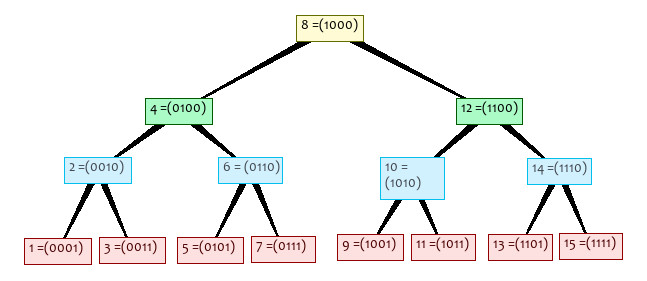2. Now if we XOR the values of the nodes, the first bit from the left set, indicates the difference in path, so we rewrite 'matching bits', add bit of height (if you have not noticed, rightmost bit set in node's number is height), and fill the rest with 0's exampli gratia:
1101 XOR 1111 = 0010 that gives us: 1110
1101 XOR 1011 = 0110 that gives us: 1100
matched bits are marked with green colour.
3. Everything would be cool and simple, but if we take two nodes on the same path to root:
0100 XOR 0111 = 0011 that gives us: 0110 WRONG!
4. ### Wrong way

Let's add preordered numbering using DFS:Definition - Ancestor
Let G(v) be the dfs preorder number of node v, and
let s(v) be the number of nodes in subtree with root in v.
Node v is ancestor of node u iff:
G(v) <= G(u) AND G(v) + s(v) > G(u)
exampli gratia:
(nodes 4 and 8) 2 < 8 AND 2 + 7 > 8
(nodes 4 and 3) 2 < 5 AND 2 + 7 > 5
So to get LCA of two nodes, we should first make checks like above, and if it is not true, use the XOR as presented above.

## Lowest Common Ancestor - GiM's modification

1. ### GiM's simple lca-trick

First of all, it is obvious, that LCA(v, v) = v, for every node v.
Definition - Height of a node
Height of a node v is the longest path from v to leaf.
As I have mentioned earlier, the position of rightmost bit set is height of a node exampli gratia: 0001 - 0, 0111 - 0, 0100 - 2, 1000 - 3
Let v be the node with lower inorder number than u.
Our check looks like this:
if (v is even AND u < v + 2^(height(v)))
v is ancestor of u!

Small explanation. We could add second condition:
if (v is odd AND v > u - 2^(height(u)))
u is ancestor of v
but you must notice that only 'right edges' are problematic ones (the ones that xor is not working with them).2. ### Semi-proof of corectness

Since we have assumed, that v is the node with lower inorder number than u, and that we are dealing only with 'right edges', check weather v is even is equivalent to:
G(v) <= G(u)
part. Now, it is obvious that number of subnodes in full binary tree is equal to:
s(v) = 2^(height(v)+1) - 1   exampli gratia: (node 14, height 1) s(14) = 2^(2) - 1 = 3
Now 2^height(v) is simply border, that means minimum and maximum number of subnodes of node v are between: (v - 2^(height(v), v + 2^(height(v))   exampli gratia: (node 14) (14 - 2^1, 14 + 2^2) = (12, 16)
Therefore u < v + 2^(height(v)) part is equivalent to G(v) + s(v) > G(u)
Formal proof is left as an excercise.
3. ### Implementaion

I have written two versions of bsf, and bsr functions, that are returning position of rightmost and leftmost bit set respectively. One version makes use of x86 assembly instructions bsf and bsr, while the other is fast, loop unrolled version for RISC processors family (they can be used on CISC processors too of course).
Implementation is 32bit oriented, that means maximum tree can have depth of 32. It would probably reqire few changes to run it on 64bit capable processor.
You can take a look at htmlized verion: bsfr.c, bsfr.h (download at the bottom).
The lca_bin function as defined above is showed in test_lca.c
I've also creates small program (lca_gfx.c) using libbpng library, and generated some interesting images presented below.
4. ### Efectiveness

If we assume x86 bsf and bsr instructions works in O(1) time, the lca implementation (lca_bin) presented works also in O(1) time.
The 'RISC alternative' functions works also in theory in O(1) time (but in reality it works in O(logn) time, where n is number of bits, so it would require to add one more step in bsf, and bsr procedures to port them to 64bit processor).
5. ### Images!

#### Classic XOR#### Simple Ones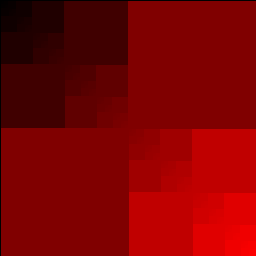row [4*i + 0] = lca_bin (i, j);
(red channel)
row [4*i + 1] = 255 - lca_bin(i, j);
(green channel)row [4*i + 2] = lca_bin (j, i);
(blue channel)

#### Reversedrow [4*i + 0] = lca_bin ((1 << BITS) - i, j);
reversed x
row [4*i + 0] = lca_bin (i, (1 << BITS) - j);
reversed y

#### Mixed channels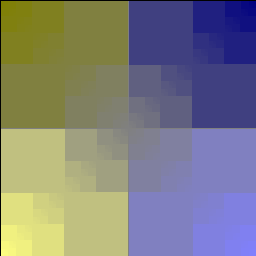row [4*i + 0] = lca_bin ((1 << BITS) - i, j);
row [4*i + 1] = lca_bin ((1 << BITS) - i, j);
row [4*i + 2] = lca_bin (i, j);

3 channels, red and green reversed x, blue normal
row [4*i + 0] = lca_bin ((1 << BITS) - i, j);
row [4*i + 1] = lca_bin (i, (1 << BITS) - j);
row [4*i + 2] = lca_bin (i, j);

3 channels, red reversed x, green reversed y, blue normal

#### 'Zoomed'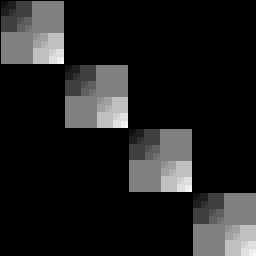row [4*i + 0] = lca_bin (i, j) >> 2;
row [4*i + 1] = lca_bin (i, j) >> 2;
row [4*i + 2] = lca_bin (i, j) >> 2;

'zoomed in'
row [4*i + 0] = lca_bin (i, j) << 2;
row [4*i + 1] = lca_bin (i, j) << 2;
row [4*i + 2] = lca_bin (i, j) << 2;

'zoomed out'

#### Most interesting

Modified version of lca_bin used to show how does interesting edges really look likerow [4*i + 0] = lca_bin_cool(i, j, 0xff);
row [4*i + 1] = lca_bin_cool(i, j, 0xff) & 0x80;

make diagonal orange, and show interesting edges (if there would be 'second condition' I've mentioned earlier, this one would result in nice square)
row [4*i + 0] = lca_bin_cool(i, j, 0);
interesting edges in black, rest normal